• 关于插值，官网有个小总结，可以直接去参考（从1维到多维），下面是我举的例子。 1, 一维插值interp1(x,y,X1,method) x = linspace(0,10,11) y = sin(x) plot(x,y,'-ro') 插值方法有如下： method=‘nearest’,...
0, 说明
关于插值，官网有个小总结，可以直接去参考（从1维到多维），下面是我举的例子。
1, 一维插值interp1(x,y,X1,method)
x = linspace(0,10,11)
y = sin(x)
plot(x,y,'-ro')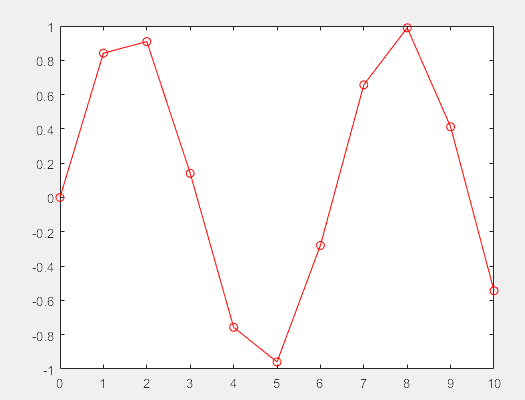插值方法有如下： method=‘nearest’,‘linear’,‘spline’,‘pchip’,‘cubic’ 比如使用三次条样插值spline，则
x = linspace(0,10,11)
y = sin(x)
plot(x,y,'-ro')

xnew = linspace(0,10,101)
f = interp1(x,y,xnew,'spline')
plot(xnew,f)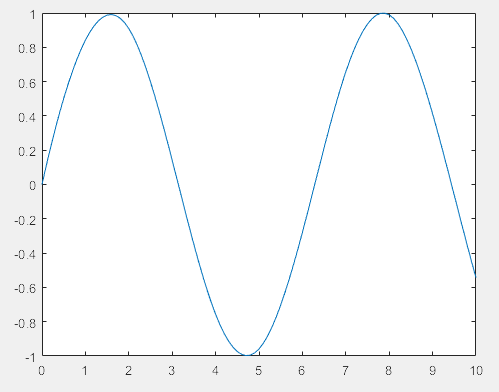2,高维插值
2.1 二维插值
使用interp2(X,Y,Z,X1,Y1,method)函数, method包含：nearest,‘linear’,‘spline’,‘cubic’
x = linspace(-5,5,11);
y = linspace(-5,5,11);
[X,Y] = meshgrid(x,y);
Z = 3.*sin(X.^2+Y.^2);

xi = linspace(-5,5,100);
yi = linspace(-5,5,100);
[XI,YI]=meshgrid(xi,yi);
ZI = interp2(X,Y,Z,XI,YI,'cubic');

surf(XI,YI,ZI),view(-25,25)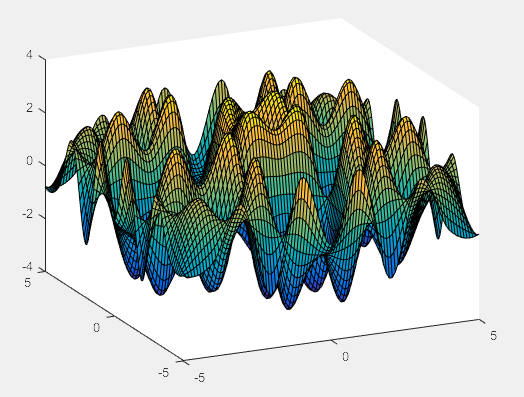2.2 三维插值
参考官网，用法如下
Vq = interp3(X,Y,Z,V,Xq,Yq,Zq)
Vq = interp3(V,Xq,Yq,Zq)

但这个方法有时候不是很实用，主要是X,Y,Z都要弄成网格的形式，因此我一般大于等于三维的时候就直接采用interpn了，见下一节。
2.3 多维插值
大于等于三维一般采用interpn,可以参考官网用法，
Vq = interpn(X1,X2,...,Xn,V,Xq1,Xq2,...,Xqn)

这里x1,x2,...xn,以及xq1,xq2,...xqn都是一维的（注意是单调函数），所以比Interp3简单很多。 方法有如下method: ‘linear’, ‘nearest’, ‘pchip’,‘cubic’, ‘makima’, or ‘spline’. The default method is ‘linear’. 举例： 1)插值一个点 现在有一个高维数据（4维），横坐标是经度，纵坐标是维度，高是海拔，V的值是在这三维中的水汽含量。我现在有了V的数据，这个数据是(37,10,10)的大小，表示高有37层，经纬度分别都是10的大小（因此经纬度构成100的数据网格），现在要计算高500m，经纬度分别为（80，32）的点的值（插值）
data_path = 'C:\Users\Lenovo\Desktop\recent work\matlab_interp\'
el = importdata([data_path,'el_sort.txt']);  %海拔
lat = importdata([data_path,'lat_sort.txt']);  %纬度
lon = importdata([data_path,'lon_sort.txt']);   %经度
Vq = interpn(el,lon,lat,V,500,80,32,'spline')    %插值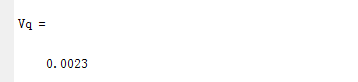2）插值两个点 上面插值只在一个点（500，80，30）上进行，但有时我们要插值的是很多个点构成的数组。
%在(500,80.03,32.3)和(900,81.2,31.4)两个点插值
Vq = interpn(el,lon,lat,V,[500 900],[80.03 81.2],[32.3 31.4],'spline')


多个点（数组）
Vq = interpn(el,lon,lat,squeeze(s_h_full(i,:,:,:)),coor(:,1),coor(:,2),coor(:,3),'spline')

这里coor表示坐标，有海拔，经，纬度，都是一列。 完整代码
data_path = 'C:\Users\Lenovo\Desktop\recent work\matlab_interp\';
el = importdata([data_path,'el_sort.txt']);  %海拔
lat = importdata([data_path,'lat_sort.txt']);  %纬度
lon = importdata([data_path,'lon_sort.txt']);   %经度
levell = 50;
hours = 720;
s_h_interp = [];
for i = 1:hours
Vq = interpn(el,lon,lat,squeeze(V(i,:,:,:)),coor(:,1),coor(:,2),coor(:,3),'spline');
s_h_interp = horzcat(s_h_interp,Vq);
end
writeNPY(s_h_interp,[data_path,'s_h_interp_matlab_161.npy'])

展开全文• ## Matlab插值计算

千次阅读 2015-04-20 10:38:55
计算插值的软件很多,这里我们只介绍如何用MATLAB做一维插值和高维插值. 4.4.1 一维插值   MATLAB中的插值函数为interp1(),其调用格式为   yi=interp1(x,y,xi, 'method') 其中x,y为观测数据点,xi为...
 计算插值的软件很多,这里我们只介绍如何用MATLAB做一维插值和高维插值.
4.4.1  一维插值
MATLAB中的插值函数为interp1(),其调用格式为
yi=interp1(x,y,xi, 'method')
其中x,y为观测数据点,xi为插值(自变量)向量,yi为xi的插值结果(函数值).'method '表示采用的插值方法.MATLAB提供的插值方法有几种:   'nearest'    最邻近插值; 'linear '线性插值; 'spline'  三次样条插值; 'cubic ' 立方插值.缺省时表示线性插值.
注意:所有的插值方法都要求x是单调的,并且xi不能够超过x的范围.
例4.2  在一天24小时内,从零点开始每间隔2小时测得的环境温度为(摄氏度)
12,9,9,10,18,24,28,27,25,20,18,15,13
推测在每一秒时的温度.并利用不同的插值方法描绘温度曲线
键入：
x=0:2:24;
y=[12 9 9 10 18 24 28 27 25 20 18 15 13];
xi=0:1/3600:24;

yi=interp1(x,y,xi,'nearest');
hold on
plot(xi,yi,'r');
yi=interp1(x,y,xi,'linear');
plot(xi,yi,'g');
yi=interp1(x,y,xi,'spline');
plot(xi,yi,'b');
yi=interp1(x,y,xi,'cubic');
plot(xi,yi,'y');还有其他的插值函数,如 interplq ,interpft, spline, intep2, interp3, interpN.
4.4.2    高维插值
N维插值函数 interpN( )
其中N可以为2,3,…,如N=2为二维插值,调用格式为
zi=interp2(x,y,z,Xi,Yi,’method’)
其中x,y为横纵坐标上的坐标点,{(x,y)}=mashgrid(x,y)生成平面网格点,z为观测到的在网格点上的二元函数值.{(x,y,z)}构成空间插值节点.引入两个向量xi,yi.xi为横坐标上的插值点,yi为纵坐标上的插值点.便可给出
[Xi,Yi]=meshgrid(xi,yi)
zi为新的或者是加细了的网格点上产生的插值结果(函数值).
'method'   表示采用的插值方法.nearest   最邻近插值,linear线性插值,cubic双三次插值.缺省时表示线性插值.所有的插值方法都要求x和y是单调的网格,x和y可以是等距的也可以是不等距的.
例如,产生一个山顶函数peaks曲面.
1)产生peaks的粗糙近似山顶曲面
[x,y,z]=peaks(10);
hold on
mesh(x,y,z)2)通过插值作出更加精细的山顶曲面
xi=-3:.1:3;
yi=xi;
[Xi,Yi]=meshgrid(xi,yi);
Zi=interp2(x,y,z,Xi,Yi,cubic);
mesh(Xi,Yi,Zi)例4.3   气旋变化情况的可视化
表4.1  是气象学家测量得到的气象资料,它们分别表示在南半球地区按不同纬度、不同月份的平均气旋数字.根据这些数据,绘制出气旋分布曲面图形.
解   下面用二维(interp2)三次(cubic)插值方法,可以得到不同月份按纬度变化的气旋值(插值结果),然后再作出其可视化图形,如图4.1MATLAB程序如下:
x=1:12;

y=5:10:85;
z1=[2.4  1.6  2.4  3.2  1.0  0.5  0.4  0.2  0.5  0.8   2.4  3.6];
z2=[18.7 21.4   16.2   9.2  2.8  1.7  1.4  2.4  5.8  9.2  10.3  16];
z3=[20.8  18.5  18.2  16.5  12.9  10.1  8.3  11.2  12.5  21.1  23.9  25.5];
z4=[22.1 20.1  20.5  25.1  29.2  32.6  33.0  31.0  28.6  32.0   28.1  25.6];
z5=[37.3  28.8  27.8  37.2  40.3  41.7  46.2  39.9  35.9  40.3  38.2  43.4];
z6=[48.2  36.6  35.5  40  37.6  35.4  35  34.7  35.7  39.5  40  41.9];
z7=[25.6  24.2  25.5  24.6  21.1  22.2  20.2  21.2  22.6  28.5  25.3  24.3];
z8=[5.3  5.3  5.4  4.9  4.9  7.1  5.3  7.3  7  8.6  6.3  6.6 ];
z9=[0.3  0  0  0.3  0  0  0.1  0.2  0.3  0  0.1  0.3];
z=[z1;z2;z3;z4;z5;z6;z7;z8;z9];
[xi,yi]=meshgrid(1:12,5:1:85);
zi=interp2(x,y,z,xi,yi,'cudic');
mesh(xi,yi,zi)
xlabel('月份'),ylabel('纬度'),zlabel('气旋'),
axis([0  12  0  90  0 50])
title('南半球气旋可视化图形')还有两个二维插值函数e01sef和e01sff,它们分别被用于求散点数据的插值函数和插值函数值,通常是两者配合使用,其调用格式为：
[fnodes,a,rnw,b,c]=e01sef(x,y,z);
[pf(i,j),ifail]=e01sff(x,y,z,rnw,fnodes,px(j),py(i);
其中x,y,z为插值节点,均为向量；px(j),py(i)为被插值点;pf(I,j)为被插值.

表4.1 南半球地区按不同纬度     不同月份的平均气旋数据

0-10      10-20      20-30     30-40     40-50     50-60     60-70     70-80     80-90
1月       2.4        18.7         20.8        22.1       37.3      48.2         25.6        5.3         0.3
2月       1.6        21.4         18.5        20.1       28.8      36.6         24.2        5.3          0
3月       2.4        16.2         18.2        20.5       27.8      35.5         25.5        5.4          0
4月       3.2         9.2          16.6        25.1       37.2      40            24.6        4.9         0.3
5月       1.0         2.8          12.9        29.2       40.3      37.6         21.1        4.9          0
6月    0.5         1.7          10.1        32.6       41.7      35.4         22.2         7.1         0
7月       0.4        1.4           8.3          33.0       46.2        35          20.2        5.3         0.1
8月       0.2        2.4          11.2         31.0       39.9      34.7         21.2        7.3         0.2
9月       0.5        5.8          12.5         28.6       35.9      35.7         22.6          7          0.3
10月   0.8        9.2          21.1        32.0       40.3      39.5         28.5         8.6          0
11月      2.4       10.3         23.9        28.1       38.2       40           25.3         6.3        0.1
12月   3.6        16           25.5         25.6      43.4       41.9        24.3         6.6         0.3
点(px(j),py(j)处的插值结果,其他输出参数涉及插值算法,可以不用了
展开全文学习 转载 编程
• 如果我们有一个基于两个向量的给定函数，参数和结果，那么我们可以找到不同参数的结果，如果它是未知的。
• 牛顿插值计算MATLAB程序 本实验主要涉及Newton插值多项式的求解。通过上机计算是学生学会程序的录入和MATLAB的使用与操作。
• 插值 插值是在已知数据之间寻找估计值的过程 一维插值 对于一维函数y=f(x)进行插值，主要分为 - 基于多项式插值 - 基于快速傅里叶插值 基于多项式插值 线性插值： 两个数据点之间的中间值都落在这两个数据点...
插值
插值是在已知数据之间寻找估计值的过程
一维插值
对于一维函数y=f(x)进行插值，主要分为  - 基于多项式插值  - 基于快速傅里叶插值
基于多项式插值
线性插值：  两个数据点之间的中间值都落在这两个数据点连成的直线上。数据点数目增多和数据点之间的距离缩短，都会使插值越来越精确。
inter1(x,y,x x,metstr)
返回长度和向量x相同的向量x x。函数f由向量x,y决定，形式y=f(x)。向量x必须按升序或者降序排列。  metstr的不同插值方法  - linear 线性插值  - nearest 最邻近插值  - spline 三次样条插值，外推法  - cubic 三次插值
对于sin($x^2$)在区间[0,2]上20个函数值的表
x = linspace(0,2,20);
y = sin(x.^2);
valuve = interp1(x,y,[0 1/2 2],'spline')
plot(x,y)
hold on
plot([0 1/2 2],valuve,'r')三次插值和样条插用于满足三阶多项式的数据之间的插值
基于快速傅里叶插值
原理：利用傅里叶变换把输入数据变换到频域，然后用更多的点进行傅里叶逆变换，结果是对数据的增采样
函数
interpft()函数
y = interpft(x,n)
对x进行傅里叶变换，然后采用n点的傅里叶逆变换回到时域。
x的长度为m,采样间隔为dx，则y的采样间隔是

dx∗m/n

d

x

∗

m

/

n

$dx*m/n$

n值必须大于m。x为矩阵，函数在x的列上，返回结果与x列数相同，行数为n的向量

0到20对于y=cos(x)进行快速傅里叶插值
%一维快速傅里叶插值实现数据增采样
x = 0:2:20;
y = cos(x);
n = 2*length(x) - 1;
yi = interpft(y,n);
xi = 0:1:20;
hold on
plot(x,y,'r-*');
plot(xi,yi,'bo-.');
legend('原始数据','插值数据');二维插值
二维插值是对于两变量函数z=f(x,y)进行插值
matlab中的二维插值函数interp2
zi = interp2(x,y,z,xi,yi)
x,y,z决定z=f(x,y)，返回值zi为xi,yi在函数f(x,y)上的值

zi = interp2(z,xi,yi)
若

z=n×m

z

=

n

×

m

$z = n \times m$，则

x=1:n

x

=

1

:

n

$x = 1:n$,

y=1:m

y

=

1

:

m

$y = 1:m$

zi = zi = interp2(z,ntimes)
在两点之间递归插值ntimes次

zi = interp2(x,y,z,xi,yi,method)
zi = interp2(x,y,z,xi,yi,method,extrapval)
method为插值方法，extropval为当数据超出原始数据范围时的一种外推方法
常用的插值方法  - 最邻近插值
method = 'nearest'

在已知数据的最邻近点设置插值点，对于插值点的数进行四舍五入。超出范围的点返回同一个NAN（Not a Number）
双线性插值 method = ‘linear’
未声明方法时，matlab的默认方法。插值点的值仅取决于最邻近的四个点的值  - 三次样条插值
method = 'spline'

采用三次样条函数来获得插值函数  - 双三次多项式插值
method = 'cubic'

例子：采用二次插值对于三维高斯型分布进行插值
[x,y] = meshgrid(-2:0.4:2);
z = peaks(x,y);
[xi,yi] = meshgrid(-1:0.2:1);
zi_nearest = interp2(x,y,z,xi,yi,'nearset');
zi_linear = interp2(x,y,z,xi,yi);
zi_spline = interp2(x,y,z,xi,yi,'spline');
zi_cubic = interp2(x,y,z,xi,yi,'cubic');
hold on;
subplot(2,3,1);
surf(x,y,z);
title('原始数据');

subplot(2,3,2);
surf(xi,yi,zi_nearest);
title('最邻近插值');

subplot(2,3,3);
surf(xi,yi,zi_linear);
title('线性插值');

subplot(2,3,4);
surf(xi,yi,zi_spline);
title('三次样条插值');

subplot(2,3,4);
surf(xi,yi,zi_cubic);
title('三次多项式插值');

figure; %新开绘图窗口

subplot(2,2,1);
contour(xi,yi,zi_nearest);
title('最邻近插值');

subplot(2,2,2);
contour(xi,yi,zi_linear);
title('线性插值');

subplot(2,2,3);
contour(xi,yi,zi_spline);
title('三次样条插值');

subplot(2,2,4);
contour(xi,yi,zi_cubic);
title('三次多项式插值');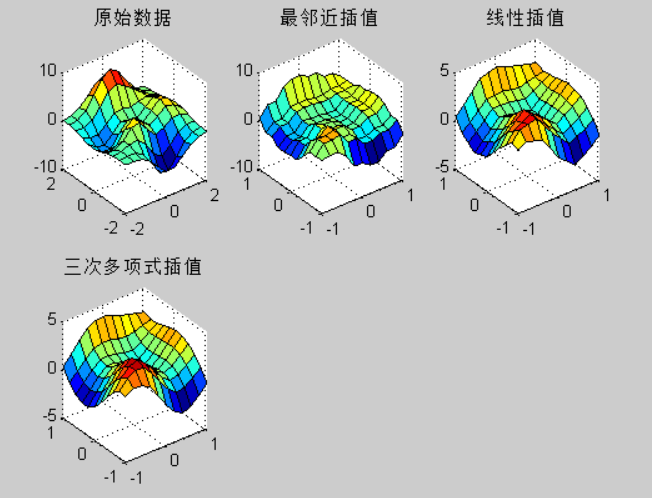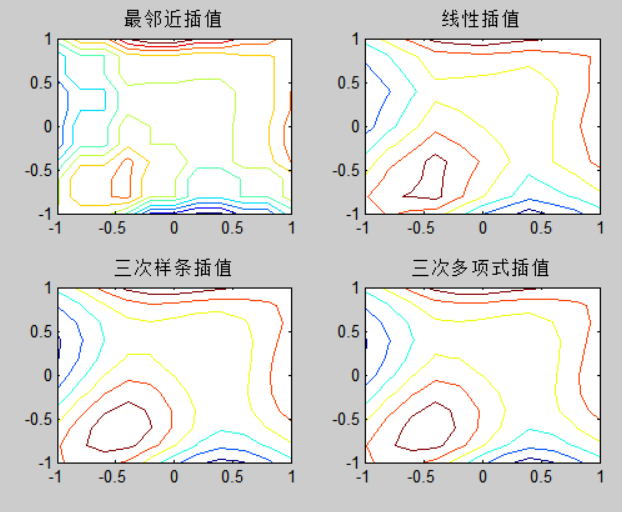特殊插值
对于没有规律的情况下的插值函数，在matlab中，可以利用delauany用于接受分散的数据点并返回一列数据的索引，用于表明各三角形的顶点。
分析一组随机数据：
x = randn(1,6);
y = randn(1,6);
t = delaunay(x,y);
hold on;
trimesh(t,x,y);
plot(x,y,'o');
hidden off;分隔区间可以用voronoi进行表示
voronoi(x,y)常用特殊插值
特殊插值表述convhull返回分散数据的凸面边界convhulln返回n维分散数据的凸面边界delaunay三角分析delaunay3三维三角分析delaunaynn维三角分析dsearch查找delaunary三角形中与某一分散点最近的点griddata二维方形栅格数据插值griddata3三维方形栅格数据插值griddatann维方形栅格数据插值tetramesh绘n维分散数据中寻找包围一个数据点的delaunay三角形voronoi对二维分散数据进行voronoi多边形分隔voronoin对n维分散数据进行voronoi多边形分隔
matlab常用插值函数
插值函数描述interp1一维插值函数interp1q一维快速插值函数interp2二维插值函数interp3三维插值函数interpft使用fft的一维插值interpnn维数据插值meshgrid产生三维函数x和y轴的索引矩阵ndgrid产生多维函数的索引矩阵
展开全文• 新乡学院数学与信息科学系 实验报告 实验项目名称 插值实验 所属课程名称 数学实验 实 验 类 型 综合性实验 实 验 日期 班 级 学 号 姓 名 成 绩 一实验概述 实验目的 掌握用Matlab插值的方法了解拉格朗日插值线性...
• matlab实现插值计算的方法，其中主要介绍了方法。希望能对您有用
• % 速度与马赫数转换函数，插值计算 % 输入:速度v 单位 m/s ，高度h 单位m 输出：Mach H=[0 100 500 1000 1500 2000 2500 3000 3500 4000 4500 5000 5500 6000 6500 7000 7500 8000 ... 8500 9000 9500 10000 11000 ...
function [Mach] = v2Mach(v,h)
% 速度与马赫数转换函数，插值计算
% 输入:速度v 单位 m/s ，高度h 单位m  输出：Mach
H=[0 100 500 1000 1500 2000 2500 3000 3500 4000 4500 5000 5500 6000 6500 7000 7500 8000 ...
8500 9000 9500 10000 11000 12000 13000 14000 15000 16000 17000 18000 19000 20000 25000 30000 ...
35000 40000 45000 50000 55000 60000 65000 70000 75000 80000]';
a=[340.3 340.0 338.4 336.4 334.5 332.5 330.6 328.6 326.6 324.6 322.6 320.5 318.5 316.5 314.4 312.3 310.2 308.1 ...
306.0 303.8 301.7 299.5 295.1*ones(1,10) 298.4 301.7 308.3 317.2 325.3 329.8 326.7 320.6 310.1 297.1 283.6 269.4]';
Vsound=interp1(H,a,h,'linear');
Mach=v/Vsound;
end

function [V] = Mach2V(Ma,h)
% 速度与马赫数转换函数，插值计算
% 输入:Mach ，高度h 单位m  输出：V 速度 单位 m/s
H=[0 100 500 1000 1500 2000 2500 3000 3500 4000 4500 5000 5500 6000 6500 7000 7500 8000 ...
8500 9000 9500 10000 11000 12000 13000 14000 15000 16000 17000 18000 19000 20000 25000 30000 ...
35000 40000 45000 50000 55000 60000 65000 70000 75000 80000]';
a=[340.3 340.0 338.4 336.4 334.5 332.5 330.6 328.6 326.6 324.6 322.6 320.5 318.5 316.5 314.4 312.3 310.2 308.1 ...
306.0 303.8 301.7 299.5 295.1*ones(1,10) 298.4 301.7 308.3 317.2 325.3 329.8 326.7 320.6 310.1 297.1 283.6 269.4]';
Vsound=interp1(H,a,h,'linear');
V=Ma*Vsound;
end

展开全文• ## matlab的插值方法

万次阅读 多人点赞 2018-08-24 21:38:28
1. Lagrange插值 插值是在已知数据之间寻找估计值的过程。在信号处理和图像处理中，插值极其常用。 类型很多：比如多项式插值，一、二、三维插值，样条插值等。 方法介绍： 对给定的n个插值点x1,x2,⋯,xnx1,x2,...
• 经常使用MATLAB的人也许会注意到MATLAB自带的一维插值函数vq = interp1(Xvec,Vvec,xq,method)调用时间过长的问题。了解他的算法可以发现，它是对xq对于Xvec向量从头到尾依次比较大小而定位到特定index的，即 for i=1...
• 以一种函数为例展示多种插值方法，包括分段线性插值、Newton插值、拉格朗日插值等等，并给出了函数文件及脚本文件
• 牛顿Newton插值法的matlab实现。给出一批离样点，做出一条通过这些点的光滑曲线，构造一个简单函数来近似。 本篇为牛顿Newton插值法，构造插值多项式。
• matlab插值算法汇总，其中包括一维，二维，曲面插值，并包括曲面法向量的计算
• Matlab插值计算各时刻磁法勘探日变观测值在磁法勘探中，消日变影响的改正称为日变改正。进行日变改正时必须设立日变站，观测日变情况。根据日变数据和测点观测时间，对观测数据进行改正。在本次磁法实习中，日变观测...
• 一物体廓线数据如下 x 0 3 5 7 9 11 12 13 14 15 y 0 1.2 1.7 2.0 2.1 2.0 1.8 1.2 1.0 1.6 用拉格朗日插值计算x每改变0.5时y的值即x 取 0.5, 1, 1.5, , 14.5 时对应的y值 程序如下 程序运行结果 > x=[0 3 5 7 9 ...
• 将下载好的工具箱解压放到matlab安装目录下的toolbox文件下，打开matlab，在set path中将dace添加到路径中。 2.教程 1）参考工具箱中的dace文档 2）https://xg1990.com/blog/archives/222 3.插值举例 1）dace...
• 本篇文章主要实现使用MATLAB进行二维插值计算 文章目录一、网格节点的插值计算二、散点数据的插值计算1. 示例 12. 示例 2 一、网格节点的插值计算 Vq = interp2(X,Y,V,Xq,Yq) 使用线性插值返回双变量函数在特定查询...数学建模
• 利用MatLab对数据进行插值计算分段线性插值三次样条插值例子 分段线性插值 应用的函数为： y=interp1(x0,y0,x)或y=interp1(x0,y0,x,’linear’) 其中的参数表示为： x0，y0表示的初始的插值节点向量 -** x表示要...
• 该例程旨在计算单位四元数，描述位于两个已知单位四元数 - q1 和 q2 之间的旋转矩阵，使用球面线性插值 - Slerp。 Slerp 遵循单位球体上最短的大圆弧，因此，可能是最短的插值路径。 因此，Slerp 具有恒定的角速度，...
• 全书正文共11章，括：MATLAB入门、线性方程组、插值、零点和根、小二乘、定积分、常微分方程、随机数、傅立叶分析、特征值和奇异值、偏微分方程。每章后都配置了大量习题。与本书正文及习题匹配的70多个M文件都由...
• ## Matlab插值方法大全

千次阅读 2018-01-11 09:10:34
该命令对数据点之间计算插值。它找出一元函数f(x)在中间点的数值。其中函数f(x)由所给数据决定。 x：原始数据点 Y：原始数据点 xi：插值点 Yi：插值点 格式 (1)yi = interp1(x,Y,xi) 返回插值向量yi，每一元素对应......

# matlab插值计算matlab 订阅